## BM25 文本相似度算法_Little Coder的博客-程序员秘密_bm25算法

#### BM25, 下一代的TF-IDF

###### 传统TF-IDF vs. BM25

BM25在传统TF-IDF的基础上增加了几个可调节的参数，使得它在应用上更佳灵活和强大，具有较高的实用性。

BM25同样使用词频，逆文档频率以及字段长度归一化，但是每个因子的定义都有细微差别

• TF-IDF没有考虑词频上限的问题，因为高频停用词已经被移除了）

• BM25 有一个上限，文档里出现5-10次的词会比那些只出现一两次的对相关度有显著影响），参见词频饱和度图：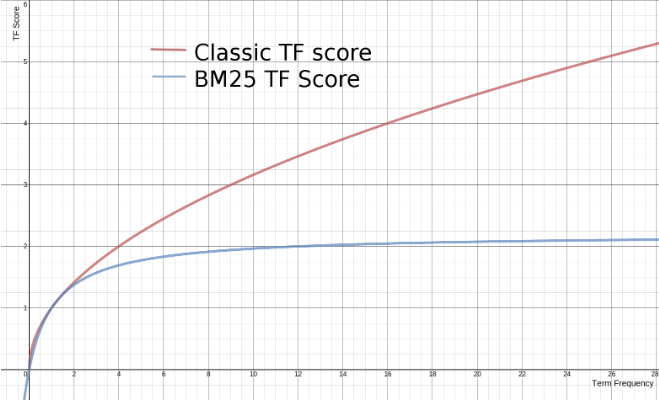BM25算法的一般性公式如下：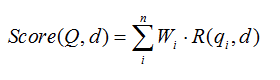• Q表示Query
• qi表示Q解析之后的一个语素（对中文而言，我们可以把对Query的分词作为语素分析，每个词看成语素qi。）
• d表示一个搜索结果文档；
• Wi表示语素qi的权重；
• R(qi，d)表示语素qi与文档d的相关性得分。
##### Wi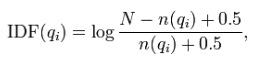• N为索引中的全部文档数(可以理解为一篇文章的所有句子数)
• n(qi)为包含了qi的文档数(可以理解为word出现在了几个句子中的句子数量)
hanlp中代码实现：
``````Map<String, Double> idf = new TreeMap<String, Double>();
idf.put(word, Math.log(D - freq + 0.5) - Math.log(freq + 0.5));
``````

##### R（qi，d）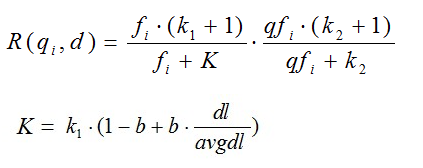• k1，k2，b为调节因子，通常根据经验设置，一般k1=2，b=0.75；
• fi为qi在d中的出现频率；（可以理解为每个word在文章中的词频）
• qfi为qi在Query中的出现频率;（可以理解为每个word在那句话中的词频）
• dl为文档d的长度;
• avgdl为所有文档的平均长度。

• k1
这个参数控制着词频结果在词频饱和度中的上升速度。默认值为 1.2 。值越小饱和度变化越快，值越大饱和度变化越慢。
• b
这个参数控制着字段长归一值所起的作用， 0.0 会禁用归一化， 1.0 会启用完全归一化。默认值为 0.75 。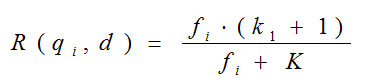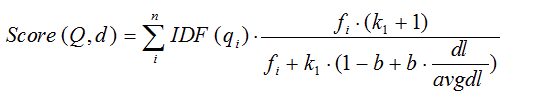hanlp中的计算代码：

``````score =  (idf.get(word) * tf * (k1 + 1)  / (tf + k1 * (1 - b + b * d  / avgdl)))
``````

``````public class BM25
{
/**
* 文档句子的个数
*/
int D;

/**
* 文档句子的平均长度
*/
double avgdl;

/**
* 拆分为[句子[单词]]形式的文档
*/
List<List<String>> docs;

/**
* 文档中每个句子中的每个词与词频
*/
Map<String, Integer>[] f;

/**
* 文档中全部词语与出现在几个句子中
*/
Map<String, Integer> df;

/**
* IDF
*/
Map<String, Double> idf;

/**
* 调节因子
*/
final static float k1 = 1.5f;

/**
* 调节因子
*/
final static float b = 0.75f;

public BM25(List<List<String>> docs)
{
this.docs = docs;
D = docs.size();
for (List<String> sentence : docs)
{
avgdl += sentence.size();
}
avgdl /= D;
f = new Map[D];
df = new TreeMap<String, Integer>();
idf = new TreeMap<String, Double>();
init();
}

/**
* 在构造时初始化自己的所有参数
*/
private void init()
{
int index = 0;
for (List<String> sentence : docs)
{
Map<String, Integer> tf = new TreeMap<String, Integer>();
for (String word : sentence)
{
Integer freq = tf.get(word);
freq = (freq == null ? 0 : freq) + 1;
tf.put(word, freq);
}
f[index] = tf;
for (Map.Entry<String, Integer> entry : tf.entrySet())
{
String word = entry.getKey();
Integer freq = df.get(word);
freq = (freq == null ? 0 : freq) + 1;
df.put(word, freq);
}
++index;
}
for (Map.Entry<String, Integer> entry : df.entrySet())
{
String word = entry.getKey();
Integer freq = entry.getValue();
idf.put(word, Math.log(D - freq + 0.5) - Math.log(freq + 0.5));
}
}

/**
* 计算一个句子与一个文档的BM25相似度
*
* @param sentence 句子（查询语句）
* @param index    文档（用语料库中的下标表示）
* @return BM25 score
*/
public double sim(List<String> sentence, int index)
{
double score = 0;
for (String word : sentence)
{
if (!f[index].containsKey(word)) continue;
int d = docs.get(index).size();
Integer tf = f[index].get(word);
score += (idf.get(word) * tf * (k1 + 1)
/ (tf + k1 * (1 - b + b * d
/ avgdl)));
}

return score;
}

public double[] simAll(List<String> sentence)
{
double[] scores = new double[D];
for (int i = 0; i < D; ++i)
{
scores[i] = sim(sentence, i);
}
return scores;
}
}

``````

### Android和PHP开发学习笔记3.1_叔叔有糖吃的博客-程序员秘密

1.PHP实际项目中的应用主要有：a) 用于后台脚本编程，即以命令行（CLI）的方式进行。b) 用于网络应用编程，即以mod_php或者fastCGI的方式执行。（LAMP框架） 2.PHP是一门“弱类型”语言，null，‘’，0，false还有空数组，在php中都是可以直接相等的。当时用全等号可以区分他们：echo "null==0:";var_dump(null==

### IMX6Q GPIO定义_weixin_34211761的博客-程序员秘密

ret = gpio_request_array(mx6q_sabresd_flexcan_gpios,                        ARRAY_SIZE(mx6q_sabresd_flexcan_gpios));static struct gpio mx6q_sabresd_flexcan_gpios[] = {        { SABRESD_CAN1_STBY, ...

### gulp打包时报错 Error: ENOENT: no such file or directory, chmod 'E:\Practice\myWorld\build\img\bg'_gulp no such file_宋大王的博客-程序员秘密

gulp打包时报错 Error: ENOENT: no such file or directory, chmod 'E:\Practice\myWorld\build\img\bg'gulp任务顺序文件目录gulpfile.jsconsolegulp任务顺序task clean 在打包之前，先清空build文件夹gulp.task('clean', d =&gt; { if (chec...